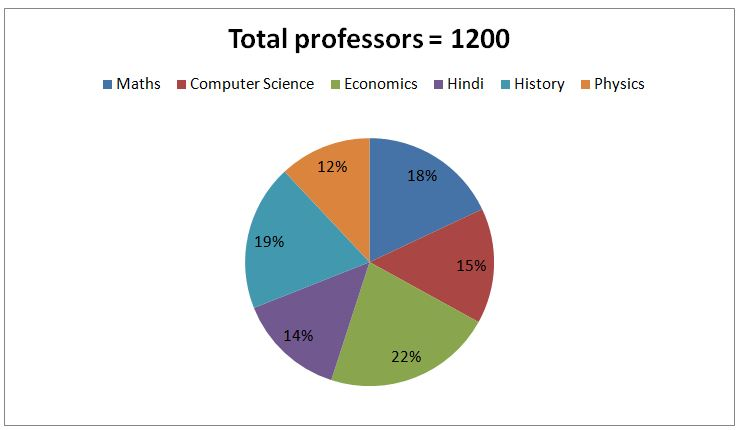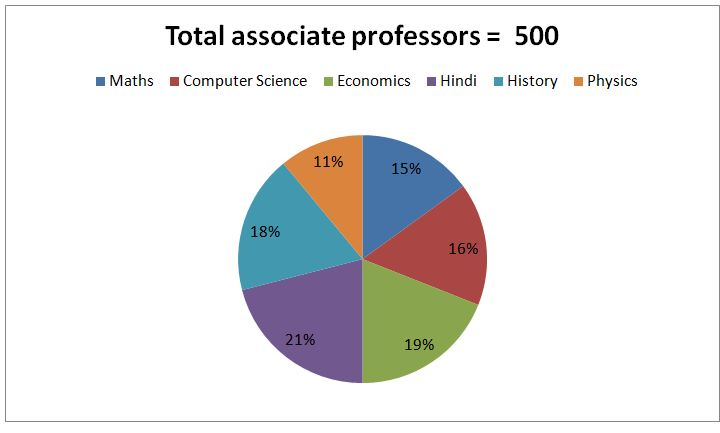# SBI PO Prelims Quantitative Aptitude Questions 2019 (Day-49)

Dear Aspirants, Our IBPS Guide team is providing new series of Quantitative Aptitude Questions for SBI PO 2019 so the aspirants can practice it on a daily basis. These questions are framed by our skilled experts after understanding your needs thoroughly. Aspirants can practice these new series questions daily to familiarize with the exact exam pattern and make your preparation effective.

[WpProQuiz 6379]

### Click Here for SBI PO Pre 2019 High-Quality Mocks Exactly on SBI Standard

Directions (Q. 1 – 5): What value should come in place of (?) in the following number series?

1) 3.5, 4.5, 11, 36, 148, ?

a) 745

b) 820

c) 685

d) 880

e) 865

2)16, 8, 12, 30, ? , 472.5

a) 96

b) 112

c) 135

d) 105

e) 154

3) 78, 56, 84, 62, ? , 68

a) 98

b) 90

c) 92

d) 94

e) 96

4) 1156, 1159, 1171, 1198, 1246, ?

a) 1378

b) 1356

c) 1321

d) 1392

e) 1415

5) 224, 113, 121, 269, 1140, ?

a) 9245

b) 8170

c) 7265

d) 9540

e) 8360

Directions (Q. 6 – 10): Study the following information carefully and answer the given questions.

The following pie chart 1 shows the percentage distribution of total number of professors (Assistant + Associate professors) teaching 6 different subjects in University A.The following pie chart 2 shows the percentage distribution of total number of Associate professors teaching 6 different subjects in University A.6) If the ratio between the total number of male professors to that of female professors in university A is 7 : 5 and the ratio between the total number of female associate professors to that of male associate professors in university A is 2 : 3, find the total number of female assistant professors in university A?

a) 450

b) 350

c) 300

d) 200

e) None of these

7) Find the difference between the assistant professors to that of associate professors of all the given subjects except the economics in university A?

a) 582

b) 126

c) 362

d) 544

e) None of these

8) Find the ratio between the total number of associate professors teaching the subjects of Maths, Economics and Hindi together to that of total assistant professors teaching the subjects of Computer science and History together?

a) 37 : 29

b) 56 : 47

c) 112 : 95

d) 275 : 238

e) None of these

9) Total number of professors teaching the subjects of Hindi and Physics together is approximately what percentage of total number of associate professors teaching the subjects of Computer science and History together?

a) 185 %

b) 155 %

c) 130 %

d) 210 %

e) 105 %

10) Find the difference between the total number of professors teaching the subjects of Maths and Economics together to that of total number of assistant professors teaching the subjects of Economics and Physics together?

a) 284

b) 316

c) 222

d) 258

e) None of these

Directions (1-5):

3.5*1 + 1 = 4.5

4.5*2 + 2 = 11

11*3 + 3 = 36

36*4 + 4 = 148

148*5 + 5 = 745

16*(1/2) = 8

8*(3/2) = 12

12*(5/2) = 30

30*(7/2) = 105

105*(9/2) = 472.5

78 – 22 = 56

56 + 28 = 84

84 – 22 = 62

62 + 28 = 90

90 – 22 = 68

(Or)

The alternative difference is, + 6

1156 + (1*3) = 1159

1159 + (2*6) = 1171

1171 + (3*9) = 1198

1198 + (4*12) = 1246

1246 + (5*15) = 1321

(Or)

The difference of difference is, 6, 6, 6,…

224*0.5 + 13 = 113

113*1 + 23 = 121

121*2 + 33 = 269

269*4 +43 = 1140

1140*8 + 53 = 9245

Directions (6-10):

Total number of female professors in university A

= > 1200*(5/12) = 500

Total number of female associate professors in university A

= > 500*(2/5) = 200

The total number of female assistant professors in university A

= >500 – 200 = 300

The average of difference between the assistant professors to that of associate professors of all the given subjects except the economics in university A

= > (141 + 100 + 63 + 138 + 89) –(75 + 80 + 105 + 90 + 55)

= > (531 – 405) = 126

The total number of associate professors teaching the subjects of Maths, Economics and Hindi together

= >500*(55/100) = 275

The total assistant professors teaching the subjects of Computer science and History together

= >[1200*(15/100) – 500*(16/100)] + [1200*(19/100) – 500*(18/100)]

= > [180 – 80] + [228 – 90]

= > 100 + 138 = 238

Required ratio = 275 : 238

Total number of professors teaching the subjects of Hindi and Physics together

= >1200*(26/100) = 312

Total number of associate professors teaching the subjects of Computer science and History together

= >500*(34/100) = 170

Required % = (312/170)*100 = 183.52 % = 185 %

The total number of professors teaching the subjects of Maths and Economics together

= >1200*(40/100) = 480

The total number of assistant professors teaching the subjects of Economics and Physics together

= >[1200*(22/100) – 500*(19/100)] + [1200*(12/100) – 500*(11/100)]

= > [264 – 95] + [144 – 55]

= > 169 + 89 = 258

Required difference = 480 – 258 = 222